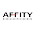## My Blog List

### C program to reverse an array

```#include
int main()
{
int n, c, d, a, b;
printf("Enter the number of elements in array\n");
scanf("%d", &n);
printf("Enter the array elements\n");
for (c = 0; c < n ; c++)
scanf("%d", &a[c]);
for (c = n - 1, d = 0; c >= 0; c--, d++)
b[d] = a[c];
for (c = 0; c < n; c++)

a[c] = b[c];

printf("Reverse array is\n");

for (c = 0; c < n; c++)

printf("%d\n", a[c]);

return 0;

}

This program reverses the array elements. For example if a is an array of integers with three elements such that
a = 1
a = 2
a = 3

Then on reversing the array will be
a = 3
a = 2
a = 1
```

1.2.